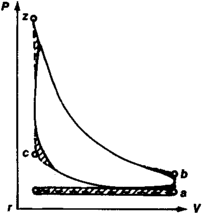# Indicator Card

Also found in: Dictionary.

## indicator card

[′in·də‚kād·ər ‚kärd]
(engineering)
A chart on which an indicator diagram is produced by an instrument called an engine indicator which traces the real-performance cycle diagram as the machine is running.
McGraw-Hill Dictionary of Scientific & Technical Terms, 6E, Copyright © 2003 by The McGraw-Hill Companies, Inc.
The following article is from The Great Soviet Encyclopedia (1979). It might be outdated or ideologically biased.

## Indicator Card

a graphic representation of changes in the pressure of a gas or vapor in the cylinder of a piston engine relative to the position of the piston. An indicator card is usually drawn by means of a pressure indicator. The volume occupied by the gases in the cylinder is plotted along the x-axis and the pressure is plotted along the y-axis. Each point on the indicator card (see Figure 1) shows the pressure in the engine cylinder at a particular volume—that is, a particular position of the piston (point r represents the start of intake; point a, the start of compression; point c, the end of compression; point z, the start of expansion; and point b, the end of expansion).Figure 1. Theoretical (dotted lines) and actual (solid lines) indicator cards for a four-cycle carburetor engine: (ra) intake line, (ac) compression line, (cz) combustion line, (zb) expansion line, (br) exhaust line; (P) pressure, (V) volume

An indicator card gives an idea of the quantity of work performed by an internal-combustion engine or a pump and of their power. The working body performs useful work only during the working stroke. Therefore, to determine useful work (efficiency) the area bounded by the compression curve ac must be subtracted from the area bounded by the expansion curve zb.

A distinction is made between theoretical and actual indicator cards. The theoretical card is plotted according to the data of thermal calculations and characterizes the theoretical cycle; the actual card is plotted by an indicator from a machine in operation and characterizes the actual cycle.

To facilitate design calculations and the comparison of various engines, pressures that change according to the piston stroke are replaced by an arbitrary constant pressure, at which work equal to the work of gases for a cycle with changing pressure is produced in a single piston stroke. The constant pressure is called the mean indicated pressure and represents the work of the gases in relation to the working volume of the engine.

B. A. KUROV